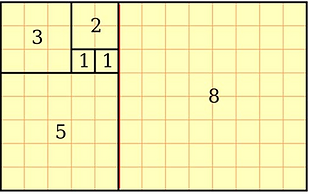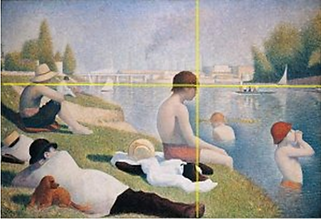## Golden Ratio

The Golden Ratio is a mathematical ratio based on the Fibonacci sequence. It is found commonly in nature and, similar to the rule of thirds, is considered to be aesthetically pleasing to the eye.

Also known as the divine proportion, the golden ratio describes a rectangle with a length roughly one and a half times its width (1.618) Many artists and architects have fashioned their works around this proportion. For example, the Parthenon in Athens and Leonardo da Vinci’s painting Mona Lisa are commonly cited examples of the ratio.

Fibonacci Sequence

The Fibonacci Sequence is the series of numbers:

0, 1, 1, 2, 3, 5, 8, 13, 21, 34, ...

The next number is found by adding up the two numbers before it.

The 2 is found by adding the two numbers before it (1+1)

• Similarly, the 3 is found by adding the two numbers before it (1+2),

• The 5 is (2+3)

and so on indefinitely!

If you divide a line into two parts so that:

the longer part divided by the smaller part

is also equal to the whole length divided by the longer part then you will have the golden ratio.Explained simply, start with a rectangle which is drawn to a ratio of 1:1.618.

If you draw a line inside the rectangle to form a perfect square, the remaining rectangle will have the same ratio as the main rectangle.

You can keep doing this over and over forever.The PanthenonThe Golden Ratio can be applied to any number of geometric forms including circles, triangles, pyramids, prisms, and polygons.

Rembrandt, Self Portrait,1640Leonardo da Vinci, Mona Lisa, c 1517George Seurat, "Bathing at Asnières", 1883-84Rule of Thirds

You may wish to view this video about the Golden Ratio

Golden Ratio